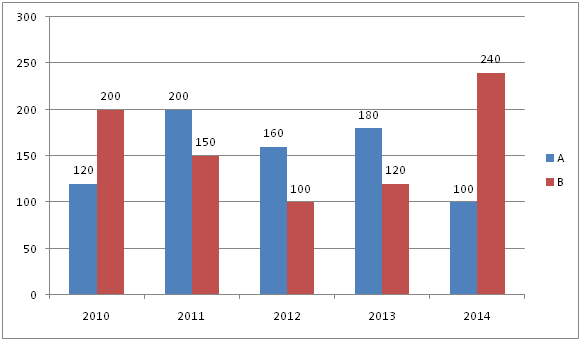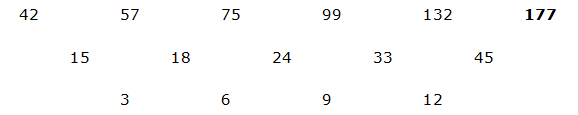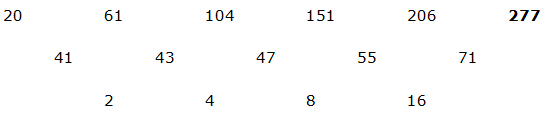# CWC/FCI Prelims 2019 – Quantitative Aptitude Questions (Day-33)

Dear Readers, Exam Race for the Year 2019 has already started, To enrich your preparation here we are providing new series of Practice Questions on Quantitative Aptitude – Section for CWC/FCI Exam. Aspirants, practice these questions on a regular basis to improve your score in aptitude section. Start your effective preparation from the right beginning to get success in upcoming CWC/FCI Exam.

[WpProQuiz 5810]

Directions (1 – 5): Find the missing number in the place of questions mark in the following series.

1) 42, 57, 75, 99, 132,?

a) 181

b) 174

c) 177

d) 149

e) 153

2) 20, 61, 104, 151, 206, ?

a) 320

b) 277

c) 420

d) 360

e) 480

3) 196, 294, 441, 661.5, ?

a) 842.25

b) 784.5

c) 1024.5

d) 992.25

e) 879.25

4) 2486, 2365, 2806, 1845, 3526, ?

a) 925

b) 845

c) 1025

d) 625

e) 125

5) 72, 73, 148, 447, 1792, ?

a) 7645

b) 8965

c) 9485

d) 6465

e) 9865

Directions (6 – 10): Study the following graph and answer the given questions

The bar graph shows the number of laptops sold in five different years by two different companies6) Number of laptops sold by A in 2010 and 2012 together is what percentage of the number of laptops sold by B in 2013 and 2014 together?

a) 66.67%

b) 55.56%

c) 77.78%

d) 88.89%

e) 84.67%

7) What is the difference between the total number of laptops sold by B in all the given years and the total number of laptops sold by A in all the given years?

a) 40

b) 30

c) 25

d) 50

e) 60

8) What is the ratio of 150% of number of laptops sold by A in 2011 and 2012 together to the 75% of number of laptops sold by B in 2012 and 2014 together?

a) 12: 13

b) 24: 25

c) 21: 25

d) 31: 33

e) None of these

9) Number of laptops sold by B in the year 2010, 2012 and 2013 together is what percentage more/less than the number of laptops sold by A in the year 2011, 2012 and 2014 together?

a) 8.69%

b) 7.64%

c) 6.59%

d) 9.56%

e) 7.25%

10) Find the average number of laptops sold by A and B in all the given years together

a) 640

b) 845

c) 785

d) 675

e) 960

Direction (1-5) :The difference of difference in the above series is 3, 6, 9, 12, 15….The pattern of the above series is *1.5, *1.5, *1.5…….

The difference of the above series is -121, +441, -961, +1681, -2601…

The pattern of the above series is *1+1, *2+2, *3+3, *4+4, *5+5…

Direction (6-10) :

Required percentage = [(120+160)/(120+240)]*100

= (280/360)*100

= 77.78%

Required difference = (200+150+100+120+240) – (120+200+160+180+100)

= 810–760

= 50

Required ratio = (150/100) * (200+160):(75/100) *(100+240)

= 2 *(360): 340

= 360: 170

= 36: 17

Required percentage

= {[(200+100+120)-(200+160+100)]/(200+160+100)}*100

= (420-460)/460*100

= (40/460)*100

= 8.69%